# 1 Introduction

This is the second part of the Bioc2016 workshop “Analysis of single-cell RNA-seq data with R and Bioconductor.”

In this part we will cover cluster analysis with the clusterExperiment package. The package is available on Github and will be in Bioconductor devel soon.

The goal of `clusterExperiment` is to encourage the user to try many different clustering algorithms in one package structure. We give tools for running many different clusterings and choices of parameters. We also provide visualization to compare many different clusterings and algorithms to find common shared clustering patterns. We implement common post-processing steps unrelated to the specific clustering algorithm (e.g. subsampling the data for stability, finding cluster-specific markers).

## 1.1 An example dataset

We will start from the normalized data obtained in the first part of the workshop with scone. The normalized data can be loaded directly from the workshop package.

``````data(scone_res)
data(ws_input)

# Summarized Experiment
se <- SummarizedExperiment(list(counts=norm_logcounts),
colData=data.frame(batch=batch[colnames(norm_logcounts)],
time_points=bio[colnames(norm_logcounts)]))``````

## 1.2 Motivation

The most common workflow for single-cell clustering found in the literature is to start from some form of normalized gene-level summaries (often on the log-scale), and perform the following steps:

1. Dimensionality reduction (usually PCA or t-SNE or most variable genes).
2. Compute a distance matrix between samples in the reduced space (usually Euclidean distance).
3. Clustering based on a partitioning method (usually k-means or PAM).

Each of these steps forces the researcher to make some choices, e.g.,

• How many principal components?
• Which distance? Euclidean, correlation, rank-based, …
• How many clusters?

These choices are very likely to impact the results.

``````pca <- prcomp(t(assay(se)), center=TRUE, scale=TRUE)
plot(pca\$x, pch=19, col=bigPalette[bio])
legend("topleft", levels(bio), fill=bigPalette)``````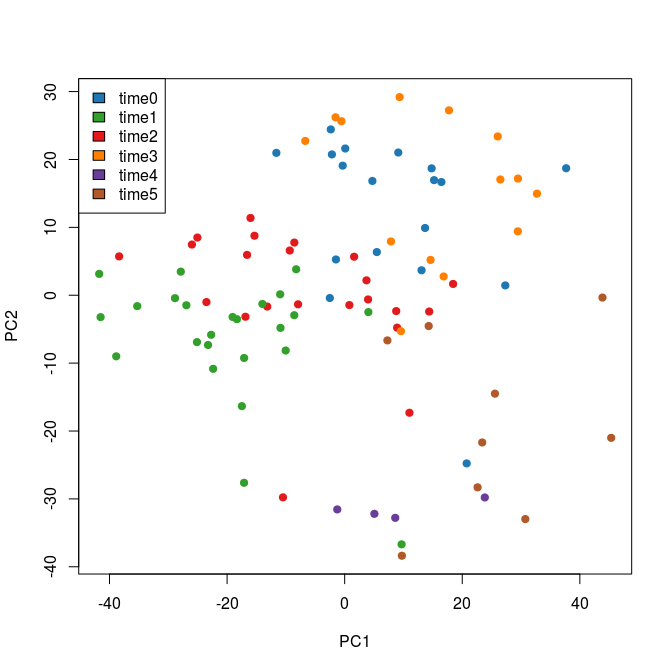``````res1 <- pam(pca\$x[,1:2], k=3)

table(res1\$clustering)``````
``````##
##  1  2  3
## 35 33 23``````
``````res2 <- pam(pca\$x[,1:3], k=3)

table(res2\$clustering)``````
``````##
##  1  2  3
## 33 27 31``````
``plot(pca\$sdev^2/sum(pca\$sdev^2), xlab="PC", ylab="Percent of explained variance")``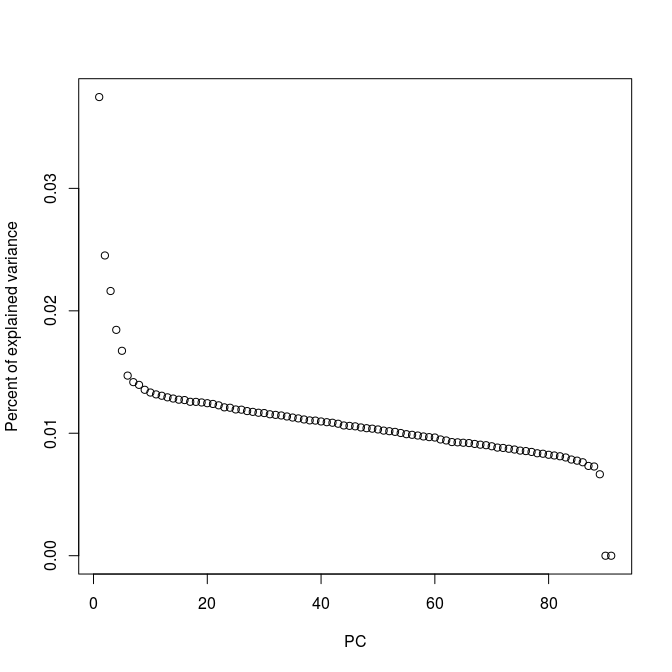``````res3 <- pam(pca\$x[,1:6], k=3)

table(res3\$clustering)``````
``````##
##  1  2  3
## 48 28 15``````

The main idea behind `clusterExperiment` (clusterExperiment) is to automatically perform and compare several clustering results, based on all possible combinations of parameters, and to find a consensus across the different clusterings.

To repeat this simple example within the `clusterExperiment` framework, we can use the function `clusterMany`.

``````cm <- clusterMany(se, dimReduce="PCA",  nPCADims=c(2, 3, 6),
ks = 3, clusterFunction = "pam")
cm``````
``````## class: ClusterExperiment
## dim: 10610 91
## Primary cluster type: clusterMany
## Primary cluster label: nPCAFeatures=2
## Table of clusters (of primary clustering):
##  1  2  3
## 35 33 23
## Total number of clusterings: 3
## No dendrogram present
## -----------
## Workflow progress:
## clusterMany run? Yes
## combineMany run? No
## makeDendrogram run? No
## mergeClusters run? No``````
``apply(clusterMatrix(cm), 2, table)``
``````##   nPCAFeatures=2 nPCAFeatures=3 nPCAFeatures=6
## 1             35             33             48
## 2             33             27             28
## 3             23             31             15``````

One of the main features of the package is the ease of visualization: For instance, we can directly compare the three results with `plotClusters`.

``````defaultMar <- par("mar")
plotCMar <- c(1.1,8.1,4.1,1.1)
par(mar=plotCMar)

plotClusters(cm)``````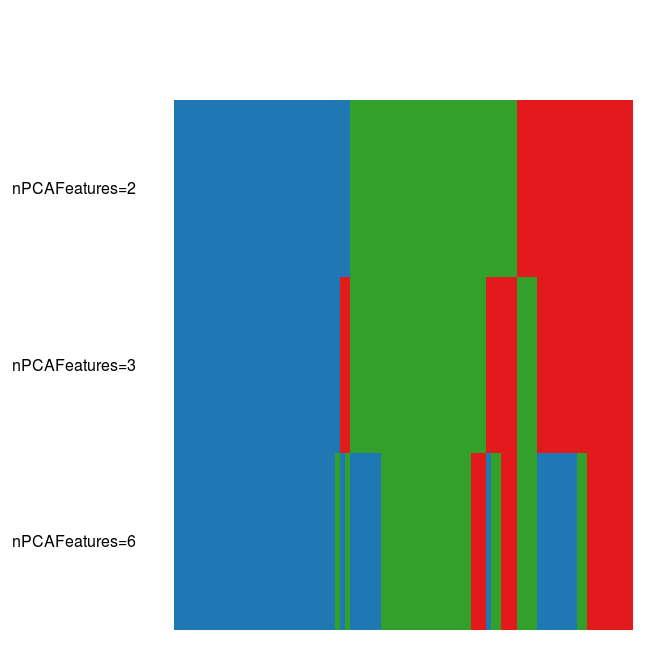We can also find a consensus across the different choices.

``cm <- combineMany(cm)``
``````## Warning in combineMany(cm): no clusters specified to combine, using results
## from clusterMany``````
``plotClusters(cm)``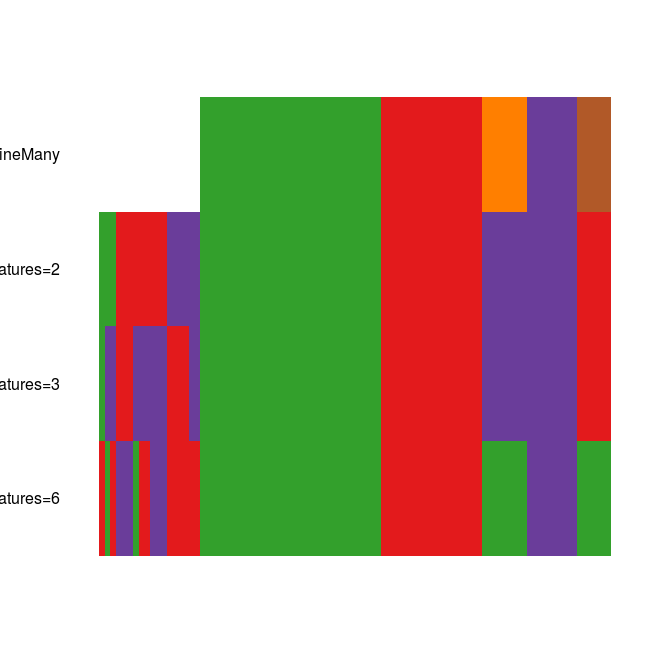``cm``
``````## class: ClusterExperiment
## dim: 10610 91
## Primary cluster type: combineMany
## Primary cluster label: combineMany
## Table of clusters (of primary clustering):
## -1 c1 c2 c3 c4 c5
## 18 32 18  8  9  6
## Total number of clusterings: 4
## No dendrogram present
## -----------
## Workflow progress:
## clusterMany run? Yes
## combineMany run? Yes
## makeDendrogram run? No
## mergeClusters run? No``````

Notice that samples are now marked as `-1`’s to indicate that these are unclustered samples. In this case, we obtain such samples because of the default option `miSize=5` which discards all the clusters with less than 5 samples.

Note that, unlike each individual call to `pam`, we do not obtain `k = 3` clusters. In general, `combineMany` results in a larger number of smaller clusters, that can then be merged with the `mergeClusters` function.

The basic premise of our workflow is to find small, robust clusters of samples, and then merge them into larger clusters as relevant. We find that many algorithmic methods for choosing the appropriate number of clusters err on the side of too few clusters. However, we find in practice that we tend to prefer to err on finding many clusters and then merging them based on examining the data.

# 2 RSEC: Resampling-based Sequential Ensemble Clustering

The main use of the package is to apply the RSEC algorithm to single-cell RNA-seq data.

The idea behind RSEC is to find a large number of small, coherent clusters by:

• Subsampling of data to find robust clusters.
• Perform sequential clustering to find a group of coherent samples, remove them, start over.

We perform this routine over many parameters and find a single consensus.

## 2.1 The `RSEC` workflow

The basic intended clustering workflow is implemented in the `RSEC` wrapper function and consists of the following steps (also available as individual functions).

• Apply many different clusterings using different choices of parameters using the function `clusterMany`. This results in a large collection of clusterings, where each clustering is based on different parameters.
• Find a unifying clustering across these many clusterings using the `combineMany` function.
• Determine whether some clusters should be merged together into larger clusters. This involves two steps:
• Find a hierarchical clustering of the clusters found by `combineMany` using `makeDendrogram`
• Merge together clusters of this hierarchy based on the percentage of differential expression, using `mergeClusters`.

## 2.2 Subsample clustering

Given an underlying clustering strategy, e.g., k-means or PAM with a particular choice of K, we repeat the following:

• Subsample the data, e.g. 70% of samples.
• Find clusters on the subsample.
• Create a co-clustering matrix D: % of subsamples where samples were in the same cluster.

We can use the function `clusterSingle` to perform this strategy over a single set of parameters.

``````cl3 <- clusterSingle(se, subsample = TRUE, sequential = FALSE,
clusterFunction = c("hierarchical01"),
clusterDArgs = list(minSize=5, alpha=0.3),
subsampleArgs = list("k"=3,"clusterFunction"="pam"),
dimReduce = c("PCA"), ndims=10)

plotCoClustering(cl3)``````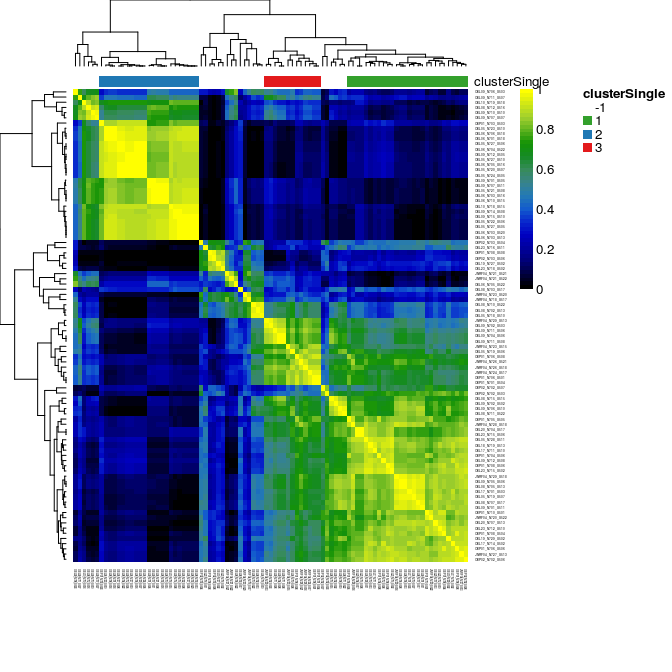``````cl7 <- clusterSingle(se, subsample = TRUE, sequential = FALSE,
clusterFunction = c("hierarchical01"),
clusterDArgs = list(minSize=5, alpha=0.3),
subsampleArgs = list("k"=7,"clusterFunction"="pam"),
dimReduce = c("PCA"), ndims=10)

plotCoClustering(cl7)``````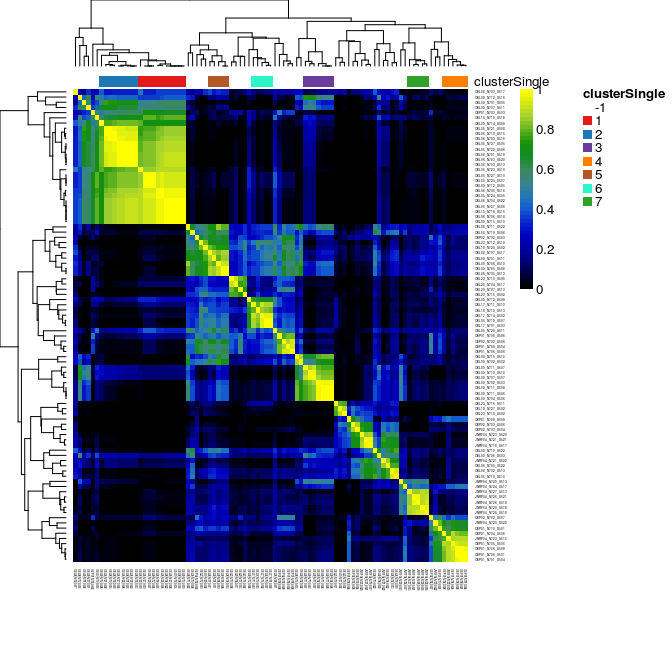Note that the final clustering is performed on the matrix D. This can be done with any clustering method. We use a flexible approach of hierarchical clustering and picking clusters so have at least 1-alpha similarity. Selecting a value for alpha seems more intuitive than selecting a number of desired clusters k.

## 2.3 Sequential clustering

Our sequential clustering works as follows.

• Range over K in PAM clustering using the subsampling strategy outlined above.
• The cluster that remains stable across values of k is identified and removed.
• Repeat until no more stable clusters are found.

This strategy draws ideas from the “tight clustering” algorithm used to find groups of genes in microarray data in (Tseng and Wong 2005).

Again, one can use the `clusterSingle` function to perform sequential clustering.

``````cl <- clusterSingle(se, subsample = TRUE, sequential = TRUE,
clusterFunction = c("hierarchical01"),
clusterDArgs = list(minSize=5, alpha=0.3),
subsampleArgs = list("clusterFunction"="pam"),
seqArgs = list(k0=5, remain.n=10, top.can=5),
dimReduce = c("PCA"), ndims=10)``````
``````## Number of points: 91     Dimension: 10
## Looking for cluster 1 ...
## k = 5
## k = 6
## Cluster 1 found. Cluster size: 6     Remaining number of points: 85
##  Looking for cluster 2 ...
## k = 4
## k = 5
## Cluster 2 found. Cluster size: 10    Remaining number of points: 75
##  Looking for cluster 3 ...
## k = 3
## k = 4
## Cluster 3 found. Cluster size: 21    Remaining number of points: 54
##  Looking for cluster 4 ...
## k = 3
## k = 4
## Did not find 5 clusters: found 4,4 clusters for k= 3,4 , respectively
## Cluster 4 found. Cluster size: 6     Remaining number of points: 48
##  Looking for cluster 5 ...
## k = 3
## k = 4
## Did not find 5 clusters: found 4,5 clusters for k= 3,4 , respectively
## Cluster 5 found. Cluster size: 5     Remaining number of points: 43
##  Looking for cluster 6 ...
## k = 3
## k = 4
## Did not find 5 clusters: found 3,4 clusters for k= 3,4 , respectively
## Cluster 6 found. Cluster size: 7     Remaining number of points: 36
##  Looking for cluster 7 ...
## k = 3
## k = 4
## Did not find 5 clusters: found 4,2 clusters for k= 3,4 , respectively
## k = 5
## Did not find 5 clusters: found 2,2 clusters for k= 4,5 , respectively
## Cluster 7 found. Cluster size: 8     Remaining number of points: 28
##  Looking for cluster 8 ...
## k = 3
## k = 4
## Did not find 5 clusters: found 1,1 clusters for k= 3,4 , respectively
## Cluster 8 found. Cluster size: 7     Remaining number of points: 21
##  Looking for cluster 9 ...
## k = 3
## k = 4
## Did not find 5 clusters: found 1,1 clusters for k= 3,4 , respectively
## k = 5
## Did not find 5 clusters: found 1,1 clusters for k= 4,5 , respectively
## k = 6
## Found  1,0 clusters for k= 5,6 , respectively. Stopping iterating because zero-length cluster.
## Stopped because: Stopped in midst of searching for cluster 9  because no clusters meeting criteria found for iteration k= 6 and previous clusters not similar enough.``````
``cl``
``````## class: ClusterExperiment
## dim: 10610 91
## Primary cluster type: clusterSingle
## Primary cluster label: clusterSingle
## Table of clusters (of primary clustering):
## -1  1  2  3  4  5  6  7  8
## 21  6 10 21  6  5  7  8  7
## Total number of clusterings: 1
## No dendrogram present
## -----------
## Workflow progress:
## clusterMany run? No
## combineMany run? No
## makeDendrogram run? No
## mergeClusters run? No``````

## 2.4 Finding a consensus

The final ingredient is finding a consensus across parameter values. For instance, we can change the values of k0 in the sequential clustering. We could use the function `clusterMany` to perform many clusterings and then `combineMany` to find the consensus clusters. Instead, the package provides a wrapper to perform these steps, where many of the common parameters have reasonable defaults.

## 2.5 The `RSEC` function

The `RSEC` function implements the following steps:

1. Cluster analysis with `clusterMany`.
2. Find a consensus with `combineMany`.
3. Merge clusters together with `makeDendrogram` and `mergeClusters`.
``````rs <- RSEC(se, k0s = 4:15, dimReduce = "PCA", nPCADims=c(10, 20),
alphas=c(0.2, 0.3), clusterFunction="hierarchical01", betas=0.7,
combineProportion=0.5, combineMinSize=3,
dendroReduce = "mad", dendroNDims = 1000,
seqArgs = list(remain.n=10, top.can=5))``````
``## Note: Merging will be done on ' combineMany ', with clustering index 1``
``rs``
``````## class: ClusterExperiment
## dim: 10610 91
## Primary cluster type: mergeClusters
## Primary cluster label: mergeClusters
## Table of clusters (of primary clustering):
##  -1  m1 m10 m11  m2  m3  m4  m5  m6  m7  m8  m9
##  11  21   3   3   7  14   3   3   7   8   4   7
## Total number of clusterings: 50
## Dendrogram run on 'combineMany' (cluster index: 2)
## -----------
## Workflow progress:
## clusterMany run? Yes
## combineMany run? Yes
## makeDendrogram run? Yes
## mergeClusters run? Yes``````

There are many arguments to the RSEC function.

Argument Passed to Meaning
dimReduce transform Which dimensionality reduction to perform
nPCADims transform Number of PC’s to be used for the clustering
clusterFunction clusterD Function to use in the clustering of the co-clustering matrix
alphas clusterD 1 - similarity required between the clusters in the co-clustering matrix
k0s seqCluster Initial k values for the sequential strategy
betas seqCluster Stability required across parameters to determine that a cluster is stable
combineProportion combineMany Minimum proportion of times two samples should be together to be assigned to a cluster.
combineMinSize combineMany Clusters with size smaller than this will be ignored (resulting in a -1 label)
dendroReduce makeDendrogram How to reduce the dimension of the data when computing the dendrogram
dendroNDims makeDendrogram How many dimensions to use when computing the dendrogram
mergeMethod mergeClusters Method used to merge clusters
mergeCutoff mergeClusters Cutoff to merge clusters

The `plotClusters` function is a good way to get a sense of how many clusterings we tried and to visualize the consensus across parameters.

``````par(mar=plotCMar)
plotClusters(rs, main="Clusters from RSEC", axisLine=-1,
sampleData=c("time_points", "batch"))``````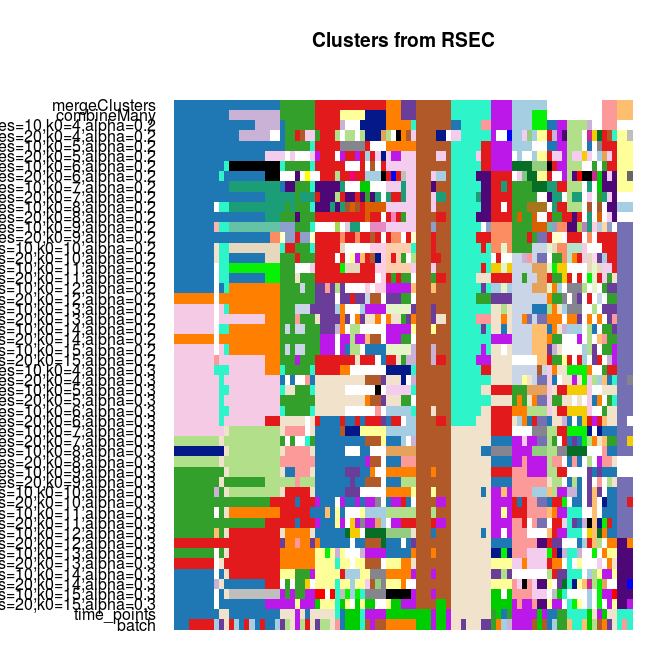This plot shows the samples in the columns, and different clusterings on the rows. Each sample is color coded based on its clustering for that row, where the colors have been chosen to try to match up clusters across different clusterings that show large overlap. Moreover, the samples have been ordered so that each subsequent clustering (starting at the top and going down) will try to order the samples to keep the clusters together, without rearranging the clustering blocks of the previous clustering/row.

We can see that some clusters are fairly stable across different choices of dimensions while others can vary dramatically. Notice that some samples are white. This indicates that they have the value -1, meaning they were not clustered.

Another good visualization is the `plotCoClustering` function, which shows how many times samples in each cluster are together across parameters.

``plotCoClustering(rs)``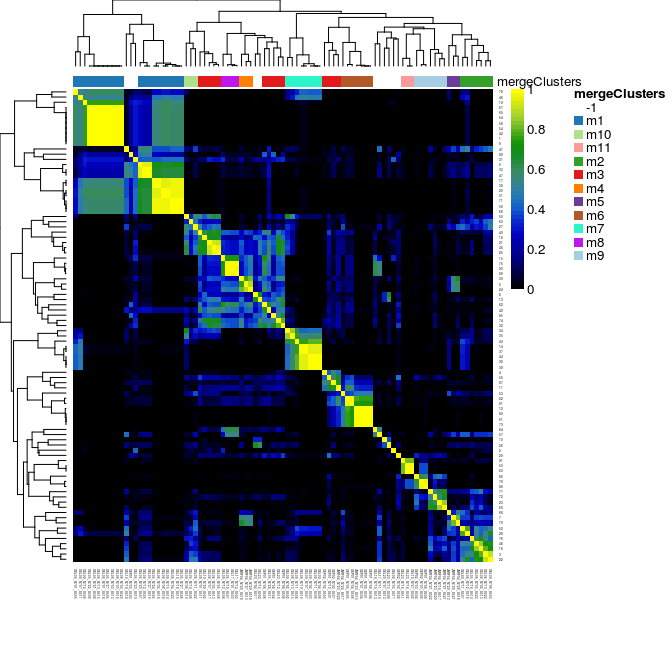To retrieve the actual results of each clustering, we can use the `clusterMatrix` and `primaryClusters` functions.

``head(clusterMatrix(rs)[,1:3])``
``````##      mergeClusters combineMany nPCAFeatures=10,k0=4,alpha=0.2
## [1,]             1           1                              1
## [2,]            -1          -1                             -1
## [3,]             2           2                              2
## [4,]             3           3                              3
## [5,]             4           4                              4
## [6,]             1           5                              5``````
``table(primaryClusterNamed(rs))``
``````##
##  -1  m1 m10 m11  m2  m3  m4  m5  m6  m7  m8  m9
##  11  21   3   3   7  14   3   3   7   8   4   7``````

## 2.6 A few details on `mergeClusters`

It is not uncommon that `combineMany` will result in too many small clusters, which in practice are too closely related to be useful. Since our final goal is to find gene markers for each clusters, we argue that we can merge clusters that show no or little differential expression (DE) between them.

This functionality is implemented in the `mergeClusters` function. `mergeClusters` needs a hierarchical clustering of the clusters; it then goes progressively up that hierarchy, deciding whether two adjacent clusters can be merged. The function `makeDendrogram` makes such a hierarchy between clusters (by applying `hclust` to the medoids of the clusters).

Here, we use the 1,000 most variable genes to make the cluster hierarchy.

``````manual <- makeDendrogram(rs, whichCluster = "combineMany", dimReduce="mad", ndims=1000)
plotDendrogram(manual)``````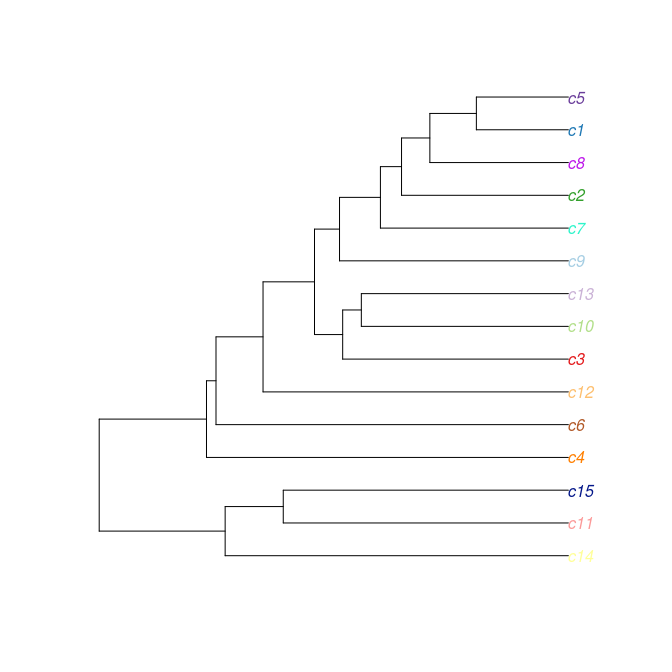It is useful to first run `mergeClusters` without actually creating any object so as to preview what the final clustering will be (and perhaps to help in setting the cutoff).

``mergeClusters(manual, mergeMethod="adjP", plot="adjP", cutoff=0.01)``
``## Note: Merging will be done on ' combineMany ', with clustering index 2``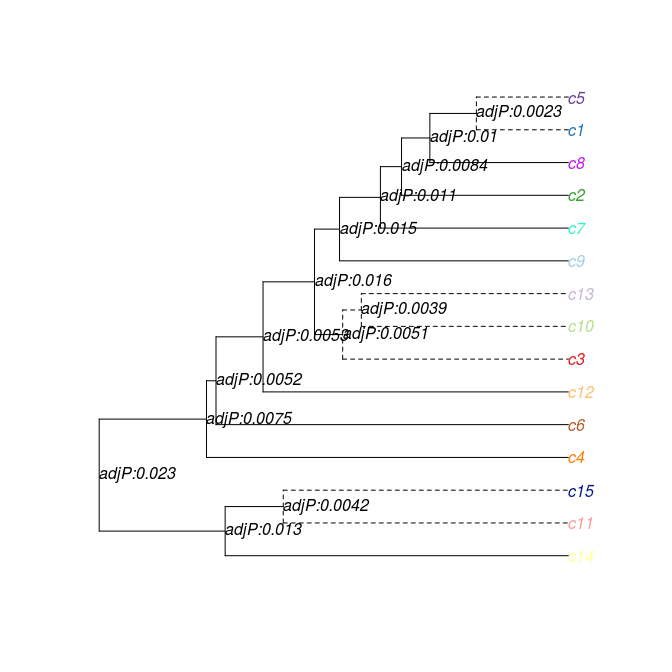``manual <- mergeClusters(manual, mergeMethod="adjP", plot="none", cutoff=0.01)``
``## Note: Merging will be done on ' combineMany ', with clustering index 2``
``````par(mar=plotCMar)
plotClusters(manual, whichClusters = c("mergeClusters", "combineMany"))
plotCoClustering(manual, whichClusters=c("mergeClusters","combineMany"))``````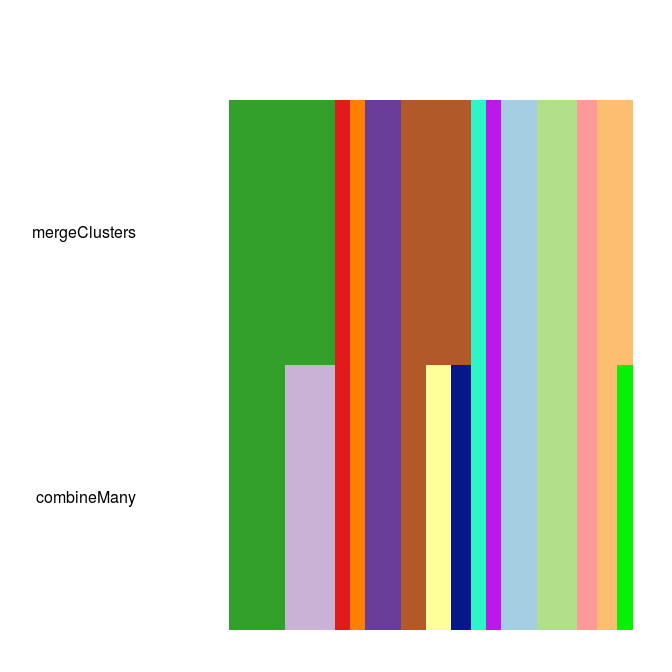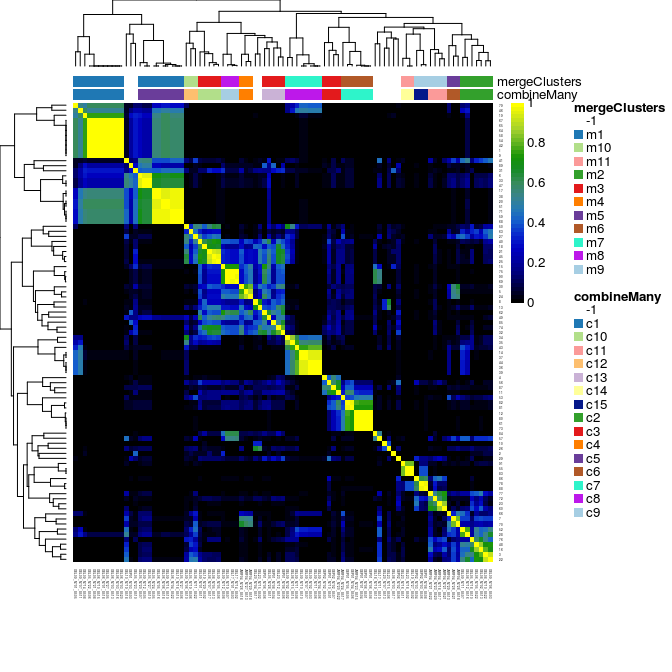Notice that `mergeClusters` combines clusters based on the actual values of the features, while the `coClustering` plot shows how often the samples clustered together.

# 3 Find marker genes with `getBestFeatures` (using limma)

Once we are satisfied with our clustering, the next step is usually to identify marker genes for each of the clusters.

The simplest way is to use differentially expressed (DE) genes to identify such markers. First, we will use `limma` as a way to compute DE genes.

When comparing multiple classes (in this case, cell types), the simplest way to identify DE genes is to look for genes DE in at least one class. This can be done using an F-test.

The utility function `getBestFeatures` uses the `lmFit` and `topTable` functions from limma to find such DE genes.

``````rs <- makeDendrogram(rs, dimReduce="mad", ndims=1000)

## set good breaks for heatmap colors
breaks <- c(min(norm_logcounts), seq(0, quantile(norm_logcounts[norm_logcounts > 0], .99, na.rm = TRUE), length = 50), max(norm_logcounts))``````
``````genesF <- getBestFeatures(rs, contrastType="F", number=500, isCount=FALSE)
``````##           IndexInOriginal   Feature X.Intercept.        cl2         cl3
## gene17844            9286 gene17844     9.168141 -0.3899279 -0.49110647
## gene6288             3307  gene6288     8.765155 -0.5046839 -0.28491910
## gene9313             4848  gene9313     7.165523 -0.0532788 -0.17263151
## gene10610            5568 gene10610     7.798969 -0.1345563 -0.57220605
## gene12034            6310 gene12034     6.523649 -0.2410174 -0.33209073
## gene12575            6607 gene12575     6.335634  0.3520281  0.03898713
##                  cl4         cl5        cl6         cl7        cl8
## gene17844 -0.7476203 -0.75258328 -0.2398202 -0.22299406  0.1076454
## gene6288  -0.3931857 -0.25096218  0.1811299  0.29617711  0.1311193
## gene9313  -0.6188552  0.10617452 -0.3630871  0.13054810 -0.2726381
## gene10610 -0.4551548 -0.33472294 -0.2554190 -0.04471818 -0.2051005
## gene12034 -0.6024025 -0.22416686 -0.5314232  0.20657270 -0.5604911
## gene12575  0.2087440  0.04471715  0.5338092  0.40055822 -0.2572012
##                  cl9        cl10       cl11  AveExpr        F      P.Value
## gene17844 -0.6885514 -0.54436921  0.1792133 8.879978 1557.694 5.602178e-84
## gene6288  -0.3366467  0.03864092  0.5077338 8.690034 1335.482 1.776294e-81
## gene9313  -0.7611721  0.24803020  0.3677072 7.035566 1222.751 4.804488e-80
## gene10610 -0.5069055  0.06878727 -0.7081651 7.552032 1217.942 5.566981e-80
## gene12034 -0.9758899  0.11446865 -0.3178064 6.266565 1103.627 2.214139e-78
## gene12575  0.4534902  0.09728133  0.5928824 6.522229 1042.196 1.880266e-77
## gene17844 5.943911e-80
## gene6288  9.423240e-78
## gene9313  1.476642e-76
## gene10610 1.476642e-76
## gene12034 4.698402e-75
## gene12575 3.324937e-74``````
``````plotHeatmap(rs, clusterSamplesData="dendrogramValue",
clusterFeaturesData=unique(genesF[,"IndexInOriginal"]),
main="F statistics",
breaks=breaks, sampleData=c("time_points"))``````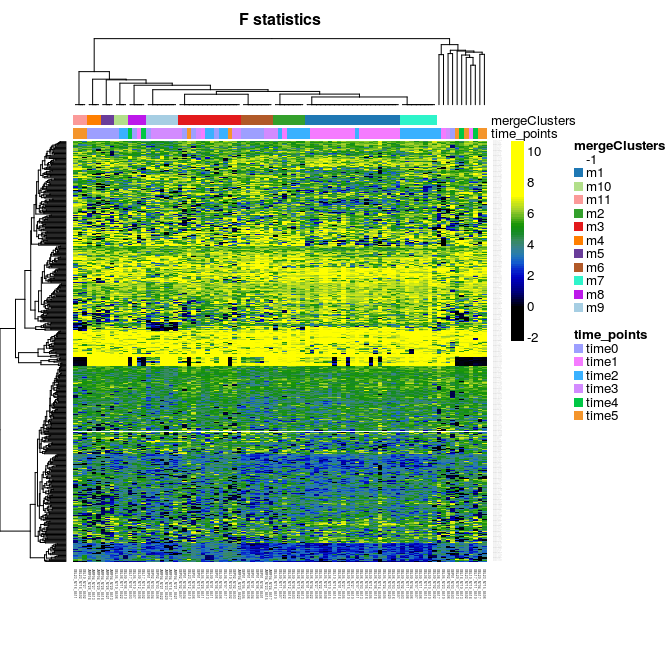The F statistic is not particularly useful to identify markers. The `getBestFeatures` function offers three alternative approaches.

• `Pairs`: finds DE genes corresponding to all pairwise comparisons.
• `OneAgainstAll`: finds DE genes comparing one cluster vs. the average of all the others.
• `Dendro`: uses the cluster hierarchy (from the dendrogram) to compute only important contrasts.
``````genesPairs <- getBestFeatures(rs, contrastType="Pairs", number=50, isCount=FALSE)

plotHeatmap(rs, clusterSamplesData="dendrogramValue",
clusterFeaturesData=unique(genesPairs[,"IndexInOriginal"]),
main="All pairwise comparisons",
breaks=breaks, sampleData=c("time_points"))``````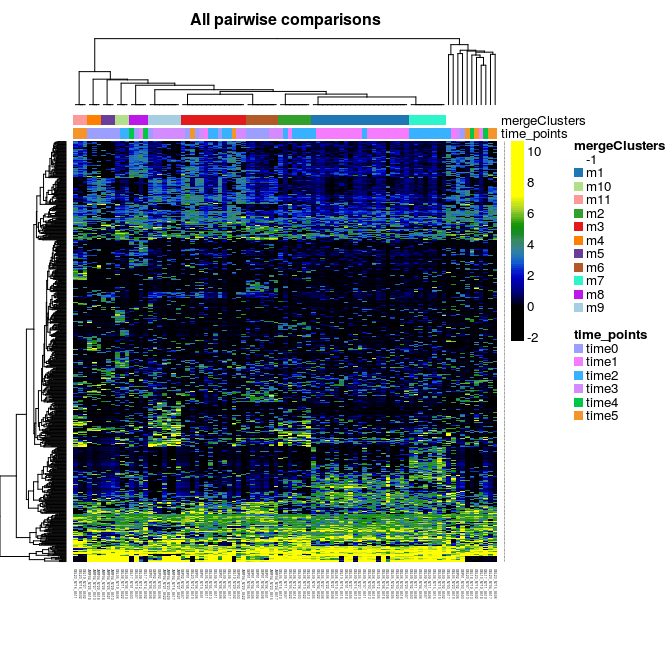``````genesOneAll <- getBestFeatures(rs, contrastType="OneAgainstAll", number=50, isCount=FALSE)

plotHeatmap(rs, clusterSamplesData="dendrogramValue",
clusterFeaturesData=unique(genesOneAll[,"IndexInOriginal"]),
main="One versus All",
breaks=breaks, sampleData=c("time_points"))``````